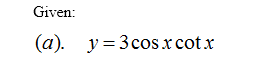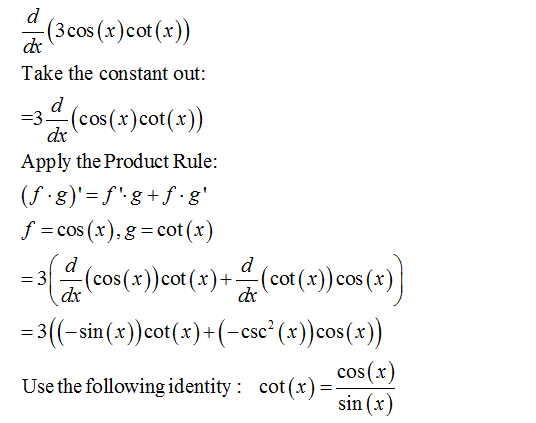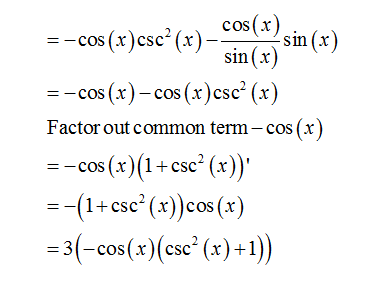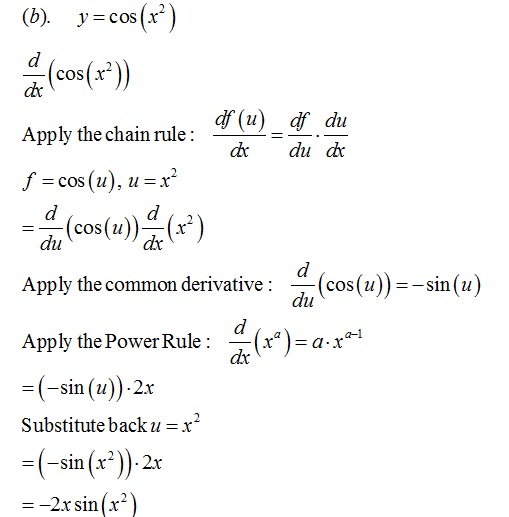# Which of the following functions can be differentiated using the rules we have covered so far? (a) y = 3 cos x cot x (b) y = cos(x2) (c) y = ex sin x

Question
1 views

Which of the following functions can be differentiated using the rules we have covered so far?
(a) y = 3 cos x cot x (b) y = cos(x2) (c) y = ex sin x

check_circle

Step 1Step 2Step 3...

### Want to see the full answer?

See Solution

#### Want to see this answer and more?

Solutions are written by subject experts who are available 24/7. Questions are typically answered within 1 hour.*

See Solution
*Response times may vary by subject and question.
Tagged in